Study Guide

# Manipulating Limits

## Manipulating Limits

Why can't limits all get along? Ignoring them is not the answer. Sometimes we need to show them who's boss by separating them, calming them down, and then bringing them back together again.

There are some nice properties of limits that allow us to evaluate limits by breaking the limits into simpler pieces.

Throughout all of these properties, a is any real number. It can be positive, negative, zero, rational, irrational, whatever, but a must be a real number.

### Basic Property 1

If c is a real number, then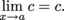Think of this as taking the limit of the constant function f(x) = c. No matter what we plug in for x, we get c as the output. If we made a table

xf(x)
x1c
x2c

every number in the f(x) column would be c. As x gets closer to a, f(x) gets closer to (or stays equal to) c.

As long as a is a real number, it doesn't even matter what a is; c is all that matters.

### Sample Problem

•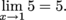•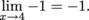•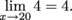### Basic Property 2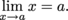Using actual numbers, what is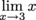?

We're looking at the limit as x approaches 3 of the function f(x) = x. If we make a table, we find

x f(x)
2.99 2.99
2.999 2.999
3.0001 3.0001
3.001 3.001
3.01 3.01

As x gets closer to 3, f(x) gets closer to 3 since x and f(x) are the same thing, So we can conclude that= 3.

There's plenty more properties to come. Stay tuned.

• ### Multiplication by a Constant

If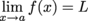,

then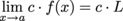.

This rule is often written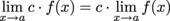.

We say that we're "pulling out'' the constant c from the limit.

### Sample Problem

For example,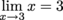and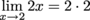.

Be Careful: This rule is only valid if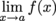is actually defined and equals L for some real number L.

We wouldn't say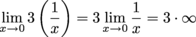because what does it mean to multiply 3 by infinity? That's like saying 3 × undefined, which doesn't make sense.

If,

is undefined (including if it equals ∞ or -∞), then the limit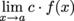is also undefined.

In pictures, if we multiply a function by a constant it means we're stretching or shrinking the function vertically. We can also stretch or shrink the limit.

For example, take the line f(x) = x and see what happens if we multiply it by 3:

As the function gets stretched, so does the limit. If we originally had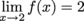then as we stretch the function by a factor of 3, the limit will also be stretched by a factor of 3: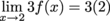If we shrink the function by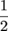, the limit will shrink by the same factor:

The limit will go fromto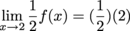Sometimes we may be asked to find a limit given partial information about a function.

For example if we're given that limxc f(x) = 4, then no matter what function f is,

limxcf(x) = 3·limxc f(x) = 3 · 4 = 12.

• ### Adding and Subtracting Limits

Limits can be added and subtracted, but only when those limits exist.

### Adding and Subtracting Property 1

If a is a real number and bothand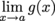exist, then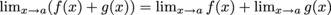In words, as long as the limits that are added both exist, "The limit of the sum is the sum of the limits.''

### Sample Problem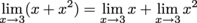= 3 + 9 = 12.

Again, "The limit of the sum is the sum of the limits".

### Sample Problem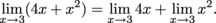By the previous rule about "pulling out'' constants,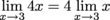= 4(3) = 12.

Therefore,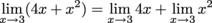= 12 + 9 = 21.

Often we use this rule in reverse. Instead of evaluating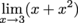by breaking it up into two limits to evaluate, we would evaluate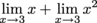by combining the limits into one.

This stuff works for subtraction, too.

### Adding and Subtracting Property 2

If a is a real number and bothandexist, then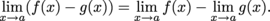In words, as long as the limits that are subtracted exist, "the limit of the difference is the difference of the limits.''

### Sample Problem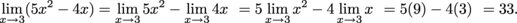Now, here's a non-example to show why we need each of the limits that are added or subtracted to exist.

### Sample Problem

Since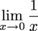does not exist, we are not allowed to say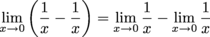We can't evaluate the left-hand side of that equation, but we can evaluate the right-hand side.

If we want to evaluate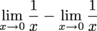we're out of luck.does not exist,

and we can't subtract "does not exist'' from "does not exist,'' since that doesn't make sense.

Infinity isn't a number, so "∞-∞"' doesn't make sense.

The point is that we can't perform the subtraction,

since evaluating the limits doesn't give us actual numbers to subtract.

Now for the right-hand side. Since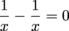,

we can easily evaluate this limit: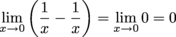The addition and subtraction rules also extend to adding several functions. as long as the limits,,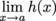all exist,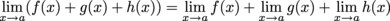### Multiplication Property

As long as bothandexist,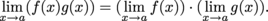In words, the limit of a product is the product of the limits, as long as the limits involved exist.

###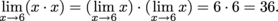Here's a few more examples of this:

• Assume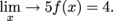Then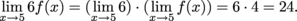• Assume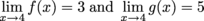Then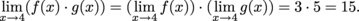• AssumeThen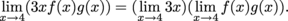### Sample Problem

Find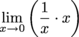If we try to break this limit into pieces, we find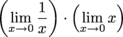This is trouble, because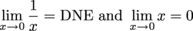What do we find if we multiply ∞ by something (if we could do such a thing, which we can't because ∞ is not a number)? It would make reasonable sense to assume we'd get ∞ again.

What do we get if we multiply a number by 0? We get 0. What if we try to multiply ∞ by 0? That makes no sense at all.

It turns out that in this case we can't find the limit of the product via the product of the limits.
However, we can still find the original limit we were asked about.

Since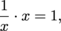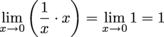### Division Property

If the limits of f and g exist and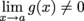,

then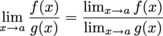Suppose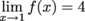and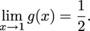Then,

•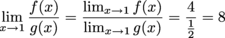•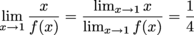•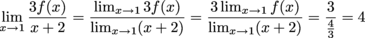If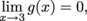, we can't use the rule we've been using to evaluate something like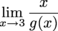.

If we try we find that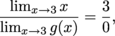then we're out of luck.

• ### Powers and Roots of Limits

Limits are pretty powerful. They're kind of the big idea of calculus. Throughout calculus we'll see that no matter what we're doing, there's a limit or two lurking somewhere.

The purpose of this reading isn't to totally fangirl over limits, though. Instead we'll be talking about what happens when we take a limit involving a function raised to some power. As it turns out, there's a property to help us with this very situation.

### Power Property

Ifexists, and p is any real number, then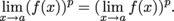The limit of a function that's being raised to some power is the limit of that function raised to the same power. All there is to it.

### Sample Problem

If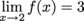then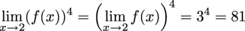Got it? Just pull the power out of the limit.